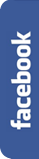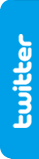# A black (and red) hole.FIRST HALFSilvio Berlusconi worries about the low number of spectators to San Siro in recent games.

After the elimination in the Champions League, and the inability to win the Scudetto (Italian League), increasingly fewer people go to the stadium to watch the matches of AC Milan.Therefore, Il Cavaliere has thought of a way to convince people to return to the stadium.

So, in the next matches, he will give away half of the gate money to one of the fans attending the match.

The lottery system is as follows:

Each person, when entering the stadium, will choose a number between 0 and 9 (inclusive), with which we'll form a number.

Thus, if the first spectator entering the stadium chooses number 1, next one picks number 4, next chooses number 3..., we will be forming the number:

1 4 3 ...

This number will have as many digits as spectators in the stadium.Once all the fans have entered, we'll have our number formed, and we will go ahead as follows:

We'll obtain a new number, whose first digits will be the amount of even digits our number has, the following figures will be the quantity of odd digits we can find in it, and finally we'll add the total number of digits (odd + even).

So, if we have number

1 4 3 5 4 8 6 9 2 3 1 2 4 3 0 7 9

The number that we will get is:

8 9 17 (8 even, 9 odd, 17 figures in total)

With this number we'll do again the same method, and thus three more times.

In total, we will proceed 5 times.The prize of half the proceeds will be given to the spectator whose seat number matches with the number resulting from this process. (All San Siro seats are numbered from 1 to 81,277).

With this draw, Berlusconi achieved an attendance success for some matches. Fans were delighted with the opportunity to take home a great prize. But gradually the attendance started to decline again.

What do you think is the reason?

## Go to the SECOND HALF to discover the solutionSECOND HALF

It seems that fans will return only when the AC Milan regain its fitness level of a few years ago. Because it's clear that the financial incentive was not enough to recover the attendance to San Siro.Specially when the list of the winners has been made public. In fact, all the awards have been delivered so far to the same spectator: Silvio Berlusconi.

How is this possible?

Let's see what happens once the stadium is filled.

We have a number of 81,277 figures:

271828182845904523536028747135266249775724709369995957496696762772407663035354

759457138217852516642742746639193200305992181741359662904357290033429526059563

073813232862794349076323382988075319525101901157383418793070215408914993488416

750924476146066808226480016847741185374234544243710753907774499206955170276183

860626133138458300075204493382656029760673711320070932870912744374704723069697

720931014169283681902551510865746377211125238978442505695369677078544996996794

686445490598793163688923009879312773617821542499922957635148220826989519366803

318252886939849646510582093923982948879332036250944311730123819706841614039701

983767932068328237646480429531180232878250981945581530175671736133206981125099

618188159304169035159888851934580727386673858942287922849989208680582574927961

048419844436346324496848756023362482704197862320900216099023530436994184914631

409343173814364054625315209618369088870701676839642437814059271456354906130310

720851038375051011574770417189861068739696552126715468895703503540212340784981

933432106817012100562788023519303322474501585390473041995777709350366041699732

972508868769664035557071622684471625607988265178713419512466520103059212366771

943252786753985589448969709640975459185695638023637016211204774272283648961342

251644507818244235294863637214174023889344124796357437026375529444833799801612

549227850925778256209262264832627793338656648162772516401910590049164499828931

505660472580277863186415519565324425869829469593080191529872117255634754639644

791014590409058629849679128740687050489585867174798546677575732056812884592054

133405392200011378630094556068816674001698420558040336379537645203040243225661

352783695117788386387443966253224985065499588623428189970773327617178392803494

650143455889707194258639877275471096295374152111513683506275260232648472870392

076431005958411661205452970302364725492966693811513732275364509888903136020572

481765851180630364428123149655070475102544650117272115551948668508003685322.......

..........................................................and so 40 more times.

There are various possibilities, as you can see:

all the spectators have chosen an odd number: 08127781277

there are less even than odd numbers, such as: 1258115281277

even and odd figures are similar: 406394063881277

there are more even numbers than odd ones, for instance: 71763951481277

everyone has chosen an even figure: 81277081277

Thus we can check that the number we get after the first process has 11 - 15 figures. In case that this number has the highest number of digits possible, that is, 15 digits, and following the same reasoning, after the second process we will get a 4 or 5 digits number:

01515, 11415, 21315, ..., 7815, ..., 15015

We choose the number with the maximum digits as possible, which is 5 digits. We apply again our method and we obtain a 3-digits number:

055, 145, 235, 325, 415, 505

After applying the same procedure a fourth time, we get a 3-digit number, which will be among the following:

033, 123, 213, 303,

In the first case: 033, if we apply the process a fourth time, we get the number 123, (1 even digit, 2 odd digits, 3 digits in total).

In the case of 123, we have the number 123 again (1 even digit, 2 odd digits, 3 digits in total).

In the case of 213, we get again 123 (1 even digit, 2 odd digits, 3 digits in total).

And in the case of 303, we will obtain also 123 (1 even digit, 2 odd digits, 3 digits in total).

So after five applications of the method, and no matter the number from which we have started, we obtain in all cases the number 123, which matches, curiously, with the seat number of Berlusconi.This is a case of 'mathematical black hole'. As in Physics we find black holes from which nothing can escape, not even light, also in Mathematics there is a similar scientific curiosity.

Thus, there are mathematical expressions and sequences of operations that always result in a numeric 'black hole' that attracts the other numbers, no matter the number you start from.

In fact, for each number we can generate a sequence of steps to convert it in a black hole.

In addition, we find black holes in arithmetic and geometric processes, and even in alphanumeric sequences. Here we have dealt with the black hole of 123 (or 213, if you write first the odd figures than the even ones), but there are many other equally curious cases.

For example, we start with any number. We write all its divisors, including 1 and itself, and we add all the digits of these divisors. With the result we go back through the same process, and so till we reach the 'black hole'. We will see that we always get number 15.

We also find the Collatz problem, which is as follows: we will build a sequence such that an+1 = an/2 if an is even, and an+1 = 3 an+1 if is odd. At the end, we always arrive at a time when the term with value 1 will be repeated forever.In English:

Even, odd and total number of digits Cut the Knot

Kaprekar constant Wikipedia

Kaprekar Wikipedia

Mysterious number 6174 Plus.maths.org - Yutaka Nishiyama)

Kaprekar routine Mathworld - Weisstein, Eric W.

Collatz conjecture Wikipedia

Another black hole number Jwilder.edublogs.org

Black hole number 15 Goodtoknowit.blogspot.com - Sunil Kumar

Mathematical black holes Recreational and educational computing - Dr. Mike Ecker

In Spanish:

Magia y agujeros negros Pedro Alegría

Constante de Kaprekar Wikipedia

Kaprekar Wikipedia

Conjetura de Collatz Wikipedia

El 123. Un agujero numérico Aprendiendomatematicas.com - Malena Martín

Una curiosa propiedad del 123 Gaussianos.com

In Catalan:

Constant de Kaprekar Wikilingua.net

Conjectura de Collatzr PuntMat.blogspot.com

If you arrived here, and want to tell us any comment on this topic, or simply suggest more links, please send us an e-mail with the following link: contact@matifutbol.com . We greatly appreciate your help, as your comments are helpful to improve our site.

If you liked our riddle, you can share it in facebook or in twitter .-->-->